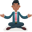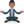Trusted answers to developer questions
Trusted Answers to Developer Questions

Related Tags

python

# What are self-powers?Abhilash

Grokking Modern System Design Interview for Engineers & Managers

Ace your System Design Interview and take your career to the next level. Learn to handle the design of applications like Netflix, Quora, Facebook, Uber, and many more in a 45-min interview. Learn the RESHADED framework for architecting web-scale applications by determining requirements, constraints, and assumptions before diving into a step-by-step design process.

### Introduction

The series where every number is raised to itself is called self-powers. For example:

11+22+33+⋯+1010=10405071317

Here, we’ll use the in-built pow() method in Python.

### The pow() method

The pow() method in python returns xy if given two arguments. It takes an optional third argument that signifies the modulo operation. If the third parameter is present, it returns xy mod z.

### Syntax

pow(x, y, z)


### Parameters

• x: It is the base number.
• y: It is the exponent number.
• z: It is the modulus number.

### Return value

The method returns the xy.

### Problem statement

Find the last ten digits of the series: 11+22+33+⋯+1010

### Solution

The most important thing to note about this issue is how quickly the terms’ sizes increase too quickly for today’s computers to be able to store them in memory. This can be avoided by making use of the fact that we only have to determine the sum’s 10 least significant digits.

We solve this problem using modular exponentiation, computing xy mod m. Modular exponentiation is achieved with the square and multiply algorithm.

Hence, the following expression:

11+22+33+⋯+1010

can be converted to

11 mod 1010 + 22 mod 1010 + 33 mod 1010 +⋯+1010 mod 1010

Now, the individual terms are restricted to 10 digits and can fit into a 64-bit word.

### Code example

Let’s look at the code below:

def solve():    target = 1000    modulus = 10 ** 10    terms = (pow(i, i, modulus) for i in range(1, target + 1))    answer = sum(terms) % modulus    return answerexpected_answer = 9110846700assert solve() == expected_answer
self powers

### Code explanation

• Line 1: We define the solve() method.

• Line 2: We define the target value.

• Line 3: We define the modulus value.

• Line 4: We calculate the individual terms of the series.

• Lines 5: We have the sum of the terms mod modulus.

• Line 9: We define the expected answer.

• Line 10: We compare the return value of the solve() method and the expected answer.

RELATED TAGS

python

CONTRIBUTORAbhilash